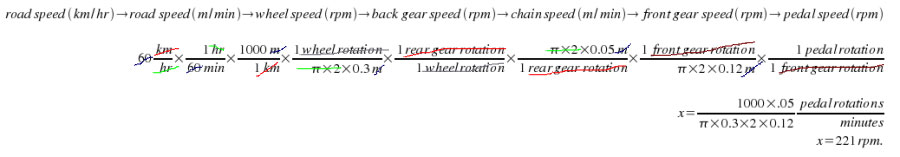SEARCH HOMEMath Central Quandaries & QueriesMatthew, a student: The pedal and gear relationship of a bicycle is shown. The radii of the gears are r(sub1) = 5 cm and r(sub2)= 12 cm. The radius of the wheel is r(sub3) = 30 cm. How many rotations per minute of the pedal gear will produce a racing cyclist's speed of 60 km per hr.Hi Matthew.

Start from the end result you want. 60 km/hr is how far per minute?

60 km/hr x (1 hr / 60 min) = 1 km / minute = 1000 m / min.

The wheel's radius is 30 cm = 0.3 m. So what is the circumference? Use π d and we get 1.885 m.

So how many times a minute must the wheel go around to achieve 1000 m / min? Do the conversion:

1000 m / min x (1 revolution / 1.885 m) = 530.5 revolutions/min = 530.5 rpm.

The gear attached to the wheel's hub moves at the same rate as the wheel itself, so the rear gear is also running at 530.5 rpm.

Since the chain is driving the rear gear, it moves the same speed as the outside of the gear. That means we look at the circumference of the gear and use that to find the chain speed. The gear has a 5cm radius, so its circumference is π (2 x 0.05 m) = 0.3142 m.

Now convert to chain speed:
530.5 rpm x (0.3142 m / revolution) = 166.67 m / minute.

The same chain is being driven by the front gear. So its circumference is π (2 x 0.12 m) = 0.7540 m.

So we can convert from chain speed in meters per minute to front gear speed in rpm:
166.67 m / minute x (1 revolution / 0.7540 m) = 221 rpm.

And since the pedals go around once for every rotation of the front gear, we are done!

There are a couple of shortcuts I could have used, but this is the right way to solve this kind of problem: work from what you know (in this case, the desired road speed in km/hr) through all the linkages. Since this is really just a bunch of conversions, we could have written it like this instead:That's less calculations. You can see how "cancelling out" units and values first makes for a lot less calculating (and rounding errors) later.

Cheers,
Stephen La Rocque.Math Central is supported by the University of Regina and The Pacific Institute for the Mathematical Sciences.